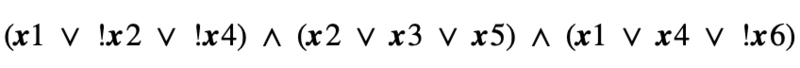# Complexity Explorer Santa Few Institute## Computation in Complex Systems (Spring 2022)

This course is no longer in session.

• Course Introduction
• Two Kinds of Paths
• Polynomials vs. Exponentials
• Divide and Conquer
• Big O and All that
• When the details don't matter
• Exam
• Divide and Conquer Redux
• Dynamic Programming
• Greedy Algorithms
• Reductions and Translations
• Lessons So Far
• The Best of All Possible Algorithms
• Complexity Wrap-Up
• Exam
• Finding versus Checking
• Circuits and Formulas
• More NP-complete Problems
• P versus NP Problem
• Existence and Nonexistence
• Above and Beyond
• Exam
• Real World Problems
• Phase Transitions
• Random Problems
• Solvability Threshold
• Modeling Differential Equations
• Landscapes, Clustering, Freezing, and Hardness
• Exam
• Building Blocks: Recursive Functions
• Building Blocks: Partial Recursive Functions
• λ Calculus
• Turing Machines
• The Halting Problem
• The Grand Unified Theory of Computation
• The Analytical Engine
• Cellular Automata
• Tile-Based Computation
• Dynamical Systems
• Exam
• More from Cris Moore
• Other ComplexityExplorer resources

#### 4.7 Quiz 4 (self-assessment) » Quiz 4: Explanation

Q1. In the Ising model interactive, why does magnetization approach zero (0) as the temperature increases past the critical temperature?

(A) The correlation between neighboring states increases with temperature

(B) The probability of that a lattice site changes increases with temperature

(C) The state of the lattice site becomes more dependent upon the system’s total energy

(D) Neighboring sites become less correlated as temperature increases

Explanation: The magnetization of a site depends on the state of its neighboring sites - however, as the temperature increases, the site is more likely to randomly change by itself. A site’s neighbors have decreasing power over its state. Part (d) is the outcome of this shift, but is not the underlying cause.

Q2. When does the transition point occur within the 3-SAT problem, where 3-SAT is easily satisfied prior to this point and difficult or impossible to solve afterwards? (Hint: See lecture 4.3)

(A) When there are more variables than clauses

(B) When the number of clauses is equal to the number of variables

(C) When variables begin to appear in a large number of clusters

(D) When there are more than five clauses

Explanation: The solvability of 3-SAT depends on the density of the clauses, or how often variables are found within each cluster. As variables appear in more clusters, the number of potential contradictions increases. The exact number depends on the number of variables and clusters. See the threshold conjunction theorem explanation in lecture 4.3 for a detailed explanation.

Q3. For values of alpha (see lecture 4.3) in the threshold conjunction theorem, which values lead to k-SAT being “difficult” to solve? Note: here, “difficult” is defined as ”taking a long time to solve”.

(A) Low values of alpha (alpha less than transition point)

(B) alpha = transition point

(C) High values of alpha (alpha greater than transition point)

Explanation: At low and high values of alpha, k-SAT is easily solvable or easily shown to have contradictions, respectively. Only when alpha is near the transition point (which changes depending on k) does k-SAT become difficult, as many possible solutions must be explored in order to find one which does not have any contradictions.

Q4. Is the following SAT problem satisfiable?(A) Yes

(B) No

Explanation: There are many possible solutions, but one case where this is satisfied is where x1 = True and x2=True. All other variables can be True or False.

Q5. Why would a transition Monte Carlo algorithm struggle as a solution landscape becomes increasingly rigid and fragmented?

(A) It is difficult for an MC algorithm to traverse across an incorrect solution space

(B) MC algorithms only can consider correct solutions

(C) A solution must be found on the first iteration of an MC algorithm

(D) MC algorithms can only work in a connected, “Mt. Fuji-like” space

Explanation: Traditional MC algorithms, also known as “hill-climbing” algorithms, consider the “correctness” of individual solutions, and iteratively choose more correct solutions in order to find the optimal solution. This becomes an issue when two or more solutions are present, but can only be found through traversing a “valley” of incorrect solutions. A traditional MC algorithm will not want to decrease the “correctness” of its solution, and therefore will stay on its local maxima. Since other solutions will not be explored, MC algorithms will struggle with a fragmented and rigid landscape.

It is worth noting that more advanced MC algorithms, such as simulated annealing - where the MC algorithm randomly jumps across the landscape at periodic intervals - can reduce this local maxima problem.